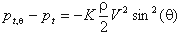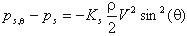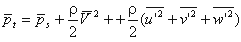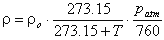• ### What are the factors influencing probe based velocity measurements in furnaces?

Date posted:

• Post Author

The factors influencing the measurement of velocity are numerous and can result in sources of error. There are, in the first instance, errors
due to the different methods of measurement, bad orientation of probe inaccuracies in the constants chosen for the probes and the
pressure transducers, non-linearity in the output of the transducers, errors in

The resultant of all these errors, which a evidently dependent upon the care
with which the measurements are taken, should not normally be more than a few percent. Other errors
can be considered as being due to difficulties in measuring conditions, i.e. turbulence of the flow, particles in suspension, the importance of molecular viscosity of the fluid at low velocities and high temperatures,
the possibility of an interaction between the pressure and the temperate both of which are required for the calculation of the velocity.

If it were possible to estimate these errors, it would require in practice a
considerable effort before a systematic measurement could be made inside a furnace The possible resultant errors can be as high as 10% for high
velocities (40 m/s) and can be more than 25% for velocities less than 4 m/s.

### 1. Reynolds Number

For low Reynolds numbers, viscous forces
are no longer negligible in comparison to inertia forces and the theorem of
Bernoulli fails to apply. This is the case for flows with Reynolds Number less
than 50. The method of Pitot remains valid provided that the measured pressure is
corrected by dividing by a factor k =
Dp/(rV2/2)
which has been calculated directly by a number of authors for simple geometric forms and within well defined Reynolds number ranges. The factor k =
Dp/(rV2/2),
with p,
r
and V being respectively the flow pressure, density and velocity, increases very rapidly when the Reynolds number is less than 50. Even though the theoretical formulae are in close agreement with experimental results it is recommended that a calibration be made for each
probe, if it is desired to achieve acceptable precision for velocities at low Reynolds numbers.

### 2. Compressibility of Gas

Bernoulli’s theorem cannot be applied when the conditions in the gas can no longer be considered as incompressible. For measurements at
high velocities the readings must be corrected. For diffusion flames in industrial furnaces, velocities are rarely higher than 80 metres per second
and therefore this problem will not be considered here.

### 3. Probe Orientation

The velocity measuring probes have directional properties. The case which has been
most investigated is that where the total pressure tapping, pt, is placed in a
spherical head or in the hemispherical head of a Prandtl probe. When
considering the pressure distribution (p
t,q)
around an obstacle, and provided that provided the angle
q
between the velocity direction and the probe is not too large, then the velocity field and consequently the pressure field is practically the same that of a potential flow, and that the error is of the formK is equal to 9/4 for a sphere and
approximately 1.9 for a hemispherical head. It has also been shown that the
static pressure, ps, around a cylinder is of the formwhere Ks depends upon the number and the
arrangement of the static pressure holes, ps,
q
and is the static pressure distribution.

### 4. Turbulence

In industrial furnaces there is a mixing
of gases at different temperatures by turbulent diffusion. The fluctuations of
velocity in magnitude and direction, particularly near the edges of the jets,
are of importance. This can be the cause of significant errors in the
measurement of velocity. Measurements are aimed in general at determining the
time mean average of the velocity which follows a relation of the form:The bar represent time mean average
quantity, and u’, v’, w’ are instantaneous values of the velocity fluctuations in
the average direction of the velocity and in two axes perpendicular to this
direction.

The basis assumption used in developing a
Classical velocity probe is that of a uniform flow field. If this is not the case then,
Bernoulli’s theorem is still applicable to the element of fluid impinging on the stagnation point. If a high degree of accuracy is required in the measurements, it is necessary to take into account that this point is not the geometrical centre of the head of the tube, but is displaced towards the position of maximum velocity. Errors can arise if the diameter D of the tube is too large and the ratio
d/D is too small.

### 5. Gas Density

In order to calculate the velocity from the pressure measurements the density of the fluid must be known, i.e. its composition, temperature and pressure. It is in
general not possible to measure all these quantities at the same time and the measurements of the physical quantities at different times can only be used if the flow remains steady. It is possible to measure the static pressure at the same time as the pressure differential. The fluctuations of temperature and composition can be very
significant in the initial region of a diffusion flame where combustion and mixing of the jets with the recirculation is taking place. These fluctuations are associated with the velocity and pressure fluctuations whose amplitude at times is greater than the mean value. The density of the gaseous mixture is given by the formula:where
ro is the density of the gas under normal conditions of 0 Celsius with pressure 760 mmHg,
patm is the atmospheric pressure at the place of the velocity measurement, and T is the temperature of the gas in Celsius.

### 6. Solid Particles in Suspension

In industrial furnaces the flames are often laden with particles. In gas and oil flames these particles are very small (mean diameter less than 1
mm), and their concentration is usually small, of the order of a few milligrammes per litre of gas under normal conditions. In pulverized coal flames the size of particles lies between 1
mm
and 200
mm and concentrations can be several grammes per litre of gas under normal conditions. These particles affect the measurements by direct deposition on the probe, blocking off the holes and burning. Account also has to be taken of changes in the density of the fluid due to the presence of the particles.

### 7. Deposition of Particles on the Probe

When measurements have been made with a Prandtl tube in a pulverized coal jet, large-scale deposition has been found on the head of the tube and under these conditions the
measurement, if it can be taken at all, is incorrect. The pressure holes, particularly the total pressure hole, block progressively, causing a damping of the fluctuations and causing a drift in the reading. The tubes have to be cleaned by blowing, through with compressed air. This operation is carried out by using a system of switches on the compressed air line by means of electro- magnetic switches. Care must be taken to protect the pressure transducer from high pressures during the blowing and after blowing: time
must be allowed for the gas in the pressure tubes to achieve equilibrium before the measurement is taken.

### 8. Probe Temperature

Temperatures, in industrial furnaces can be as high as 2000°C. Velocity measurements can be made with Water-cooled
probes. The use of these probes, however, can result in condensation of water vapour and droplets, as well as the deposition of particles. Alternatively, probes that are not water cooled have been made with refractory materials (alumina) or stainless steel, in which deposits are burnt on the probe head. At
IJmuiden, a combination is used in which a stainless steel head is left uncooled over a distance of 25 mm or 35
mm. In all cases there will be a difference in temperature between the probe and the gas. The temperature of non-cooled probes depends more on the intensity of radiation than upon the temperature of the gas. As a result of this
temperature difference there is an exchange of heat by convection between the gas and the probe, and this causes a perturbation in the boundary layer, and therefore on the
flow and its velocity.

### You may also like

• #### What is an “FTIR” analyser?

22nd March 2001

• #### What is CFD for combustion calculations?

29th April 2002